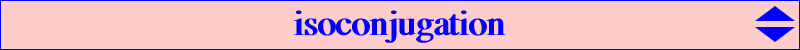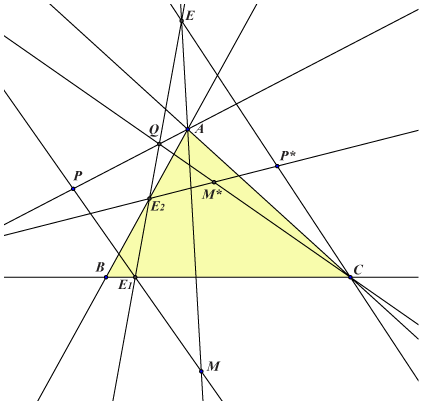Isoconjugation is a purely projective notion entirely defined with the knowledge of a pencil of conics such that the triangle ABC is self-polar with respect to any conic of the pencil. In other words, these conics are diagonal conics with respect to ABC. For any point M -- distinct from A,B,C -- the polar lines of M with respect to all the conics of the pencil are concurrent at M* (which is actually the pole of M in the pencil of conics). We call isoconjugation the mapping f : M --> M* and we say that M* is the isoconjugate of M. f is an involutive quadratic mapping with singular points A,B,C and fixed points the common points -- real or not -- of the members of the pencil. If we know two (distinct or not) isoconjugate points P and P* – provided that they do not lie on the sidelines of ABC – we are able to construct the isoconjugate M* of any point M.It is enough to construct successively (with ruler only) the following points : E = AM /\ CP*, E1 = MP /\ BC, Q = AP /\ EE1, E2 = EQ /\ AB, M* = CQ /\ P*E2. Further details (in French) here.This shows that there is no need of coordinates to define an isoconjugation. Nevertheless, when computations are needed, we will make use of barycentric coordinates and define the pole of the isoconjugation as the isoconjugate W = G* of the centroid G. This definition is now an affine notion. Hence, if W=(p:q:r), the isoconjugation with pole W is the mapping : M(x : y : z) --> M* (p y z : q z x : r x y) and then M* is said to be the W-isoconjugate of M. Remark : W is the perspector of the circum-conic which is the isoconjugate of the line at infinity. When W = K, we have the usual isogonal conjugation and when W = G , we have the isotomic conjugation. When W is inside the triangle ABC i.e. p, q, r are all positive, the W-isoconjugation has four real fixed points : one inside ABC and the three others its harmonic associates, but when W is outside the triangle ABC, the four fixed points are imaginary. The four fixed points are the square roots of W denoted Ro, Ra, Rb, Rc where Ro is the one which is inside ABC when W is itself inside the triangle.